### Introduction

The iraceplot package provides a set of functions to create plots to visualize the configuration data generated by the configuration process implemented in the irace package.

The configuration process performed by irace will show ar the end of the execution one or more configurations that are the best performing configurations found. This package provides a set of functions that allow to further assess the performance of these configurations and provides support to obtain insights about the details of the configuration process.

## Installation

### Install iraceplot from CRAN

install.packages("iraceplot")

### Install iraceplot from github

For installing iraceplot you need to install the devtools package:

install.packages("devtools")
devtools::install_github("auto-optimization/iraceplot/")

### How to use

This is a basic example that shows how to use iraceplot:

library(iraceplot)

To use the functions its required to have the log file generated by irace (logFile option in the irace package). This file is commonly saved in the in the directory in which irace was executed with the name irace.Rdata. To load the irace log data, replace the path of the file with yours:

iraceResults <- irace::read_logfile("~/path/to/irace.Rdata")

This file contains the iraceResults variable which contains the irace log. For more details about this variable, please go to the user guide of the irace package.

### Executing irace

To use the methods provided by this package you must have an irace data object, this object is saved as an Rdata file (irace.Rdata by default) after each irace execution.

During the configuration procedure irace evaluates several candidate configurations (parameter settings) on different training insrances, creating an algorithm performance data set we call the training data set. This information is thus, the data that irace had access to when configuring the algorithm.

You can also enable the test evaluation option in irace, in which a set of elite configurations will be evaluated on a set of test instances after the execution of irace is finished. Nota that this option is not enabled by default and you must provide the test instances in order to enable it. The performance obtained in this evalaution is called the test data set. This evaluation helps assess the results of the configuration in a more “real” setup. For example, we can assess if the configuration process incurred in overtuning or if a type of instance was underrepresented in the training set. We note that irace allows to perform the test evaluations to the final elite configurations and to the elite configurations of each iterations. For information about the irace setup we refer you to the irace package user guide.

Note: Before executing irace, consider setting the test evaluation option of irace.

Once irace is executed, you can load the irace log in the R console as previously shown.

## Visualizing irace configuration data

In the following, we provide an example how the functions implemented in this package can be used to visualize the information generated by irace.

### Configurations

Once irace is executed, the first thing you might want to do is to visualize how the best configurations look like. You can do this with the parallel_coord method:

parallel_coord(iraceResults)

The plot shows by default the final elite configurations (last iteration), each line in the plot represents one configuration. The plot produced by parallel_coord can help you to both the distribution of the parameter values of a set of configurations and the common associations between these values. By default, the plot colors the lines using the number of instances evaluated by the configuration. You can use the color_by_instances argument to choose between coloring the lines by the number of instances executed or the last iteration the configurationwas executed on.

To visualize the configurations considered as elites in each iteration use the iterations option. You can select one or more iterations. For example, to select all iterations executed by irace:

parallel_coord(iraceResults, iterations=1:iraceResults$state$nbIterations,
color_by_instances = FALSE)

You can also visualize all configurations sampled in one or more iterations disabling the only_elite option. For example, to visualize configurations sampled in iterations 1 to 9:

parallel_coord(iraceResults, iterations=1:9, only_elite=FALSE)

If you are looking for something more flexible and you would like to provide your own set of configurations, you can use the parallel_coord2 function. This function generates a parallel coordinates plot (similar to the ones generated by parallel_coord) when provided with an arbitrary set of configurations and a parameter space object. The configurations must be provided in the format in which irace handles configurations: a dataframe with parameter in each column. For information about these formats, please chech the irace package user guide. As an example, this lines display all elite configurations:

all_elite <- iraceResults$allConfigurations[unlist(iraceResults$allElites),]
parameters <- iraceResults$parameters parallel_coord2(all_elite, parameters) The parallel_cat function displays all configurations sampled in a set of iterations. The plot groups parameter values in intervals and thus, it can be useful to visualize more tendencies of association between parameters values. As in the previous functions you can use the iterations argument to select the iterations from which configurations should be selected. For example, to visualize configurations sampled on iterations 3, 4 and 5: parallel_cat(irace_results = iraceResults, iterations = c(3,4,5) )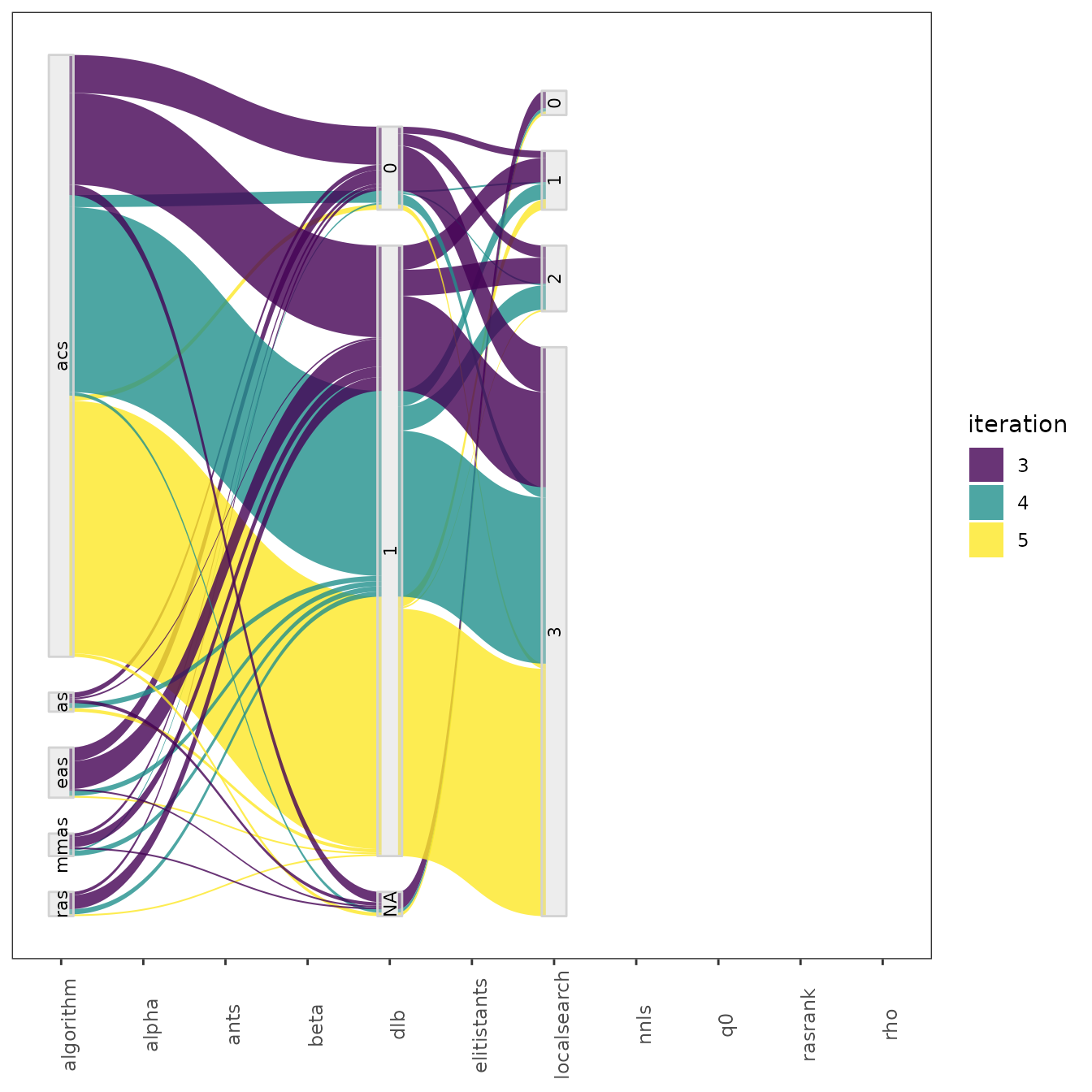You can also adjust the number of value intervals that are displayed for each parameter using the n_bins parameter which by default is set to 3. Also, it is possible to select a subset of configurations using the id_configurations argument providing a vector a configuration ids. Both in the parallel_coord and parallel_cat functions parameters can be selected using the param_names argument. For example to select parameters un parallel_cat: parallel_cat(irace_results = iraceResults, param_names=c("algorithm", "localsearch", "dlb", "nnls"))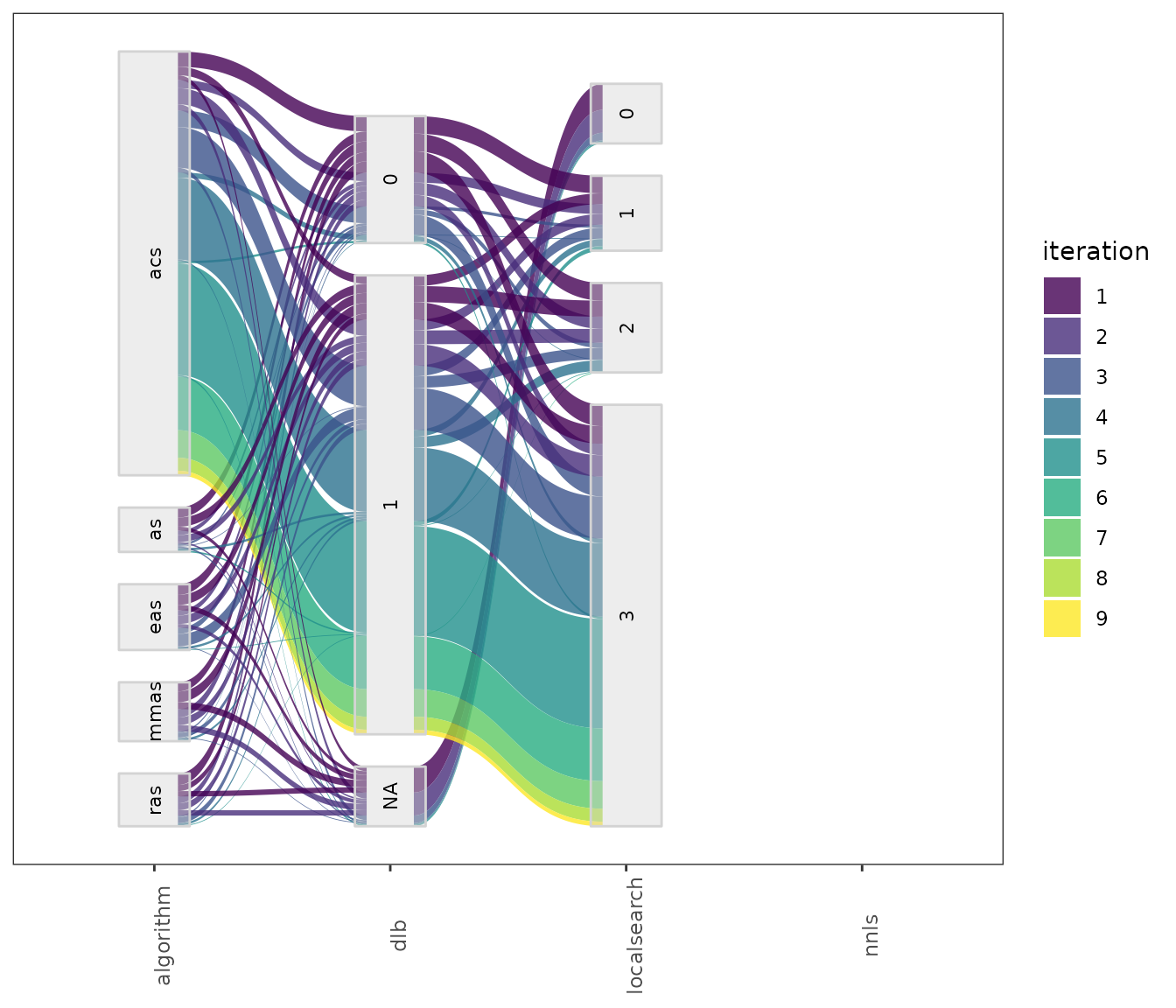This setting is useful to visualize the association between parameters that, for example, seem to interact. For configuration scenarios that define a large number of parameters, it is impossible to visualize all parameters in one of these plots. If do not know which parameters to select, all parameters can be split in different plots using the by_n_param argument which specifies the maximum number of parameters to include in a plot. The functions will generate as many plots as needed to cover all parameters included. ### Sampled values and frecuencies The sampling_pie function creates a plot that displays the values of all configurations sampled during the configuration process. This plot can be useful to display the tendencies in the sampling in a simple format. As well as in the previousparallel_cat plot, numerical parameters domains are discretized to be shown in the plot. The size of each parameter value in the plot is dependent of the number of configurations having that value in the configurations. sampling_pie(irace_results = iraceResults) As in previous plots, you can select the parameters to display with the param_names argument and the number of intervals of each parameter with the n_bins argument. The generated plot is interactive, you can click in each parameter to display it independently. In some cases in might be interesting to have a look at the values sampled during the configuration procedure as a distribution. Such plot shows the areas in the parameter space where irace detected a high performance. A general overview of the distribution of sampled parameters values can be obtained with the sampling_frequencyfunction which generates frequency and density plots for the sampled values:  sampling_frequency(iraceResults)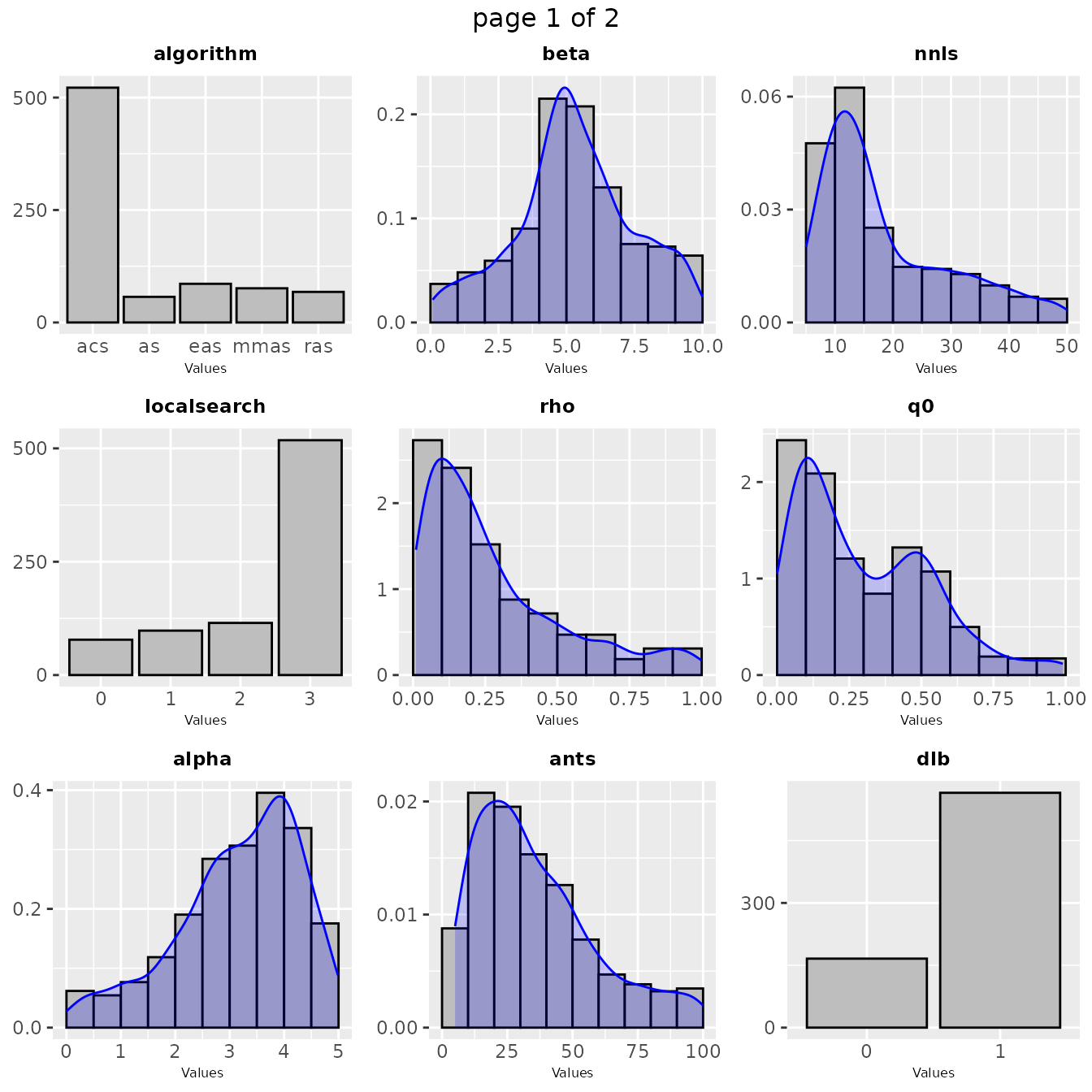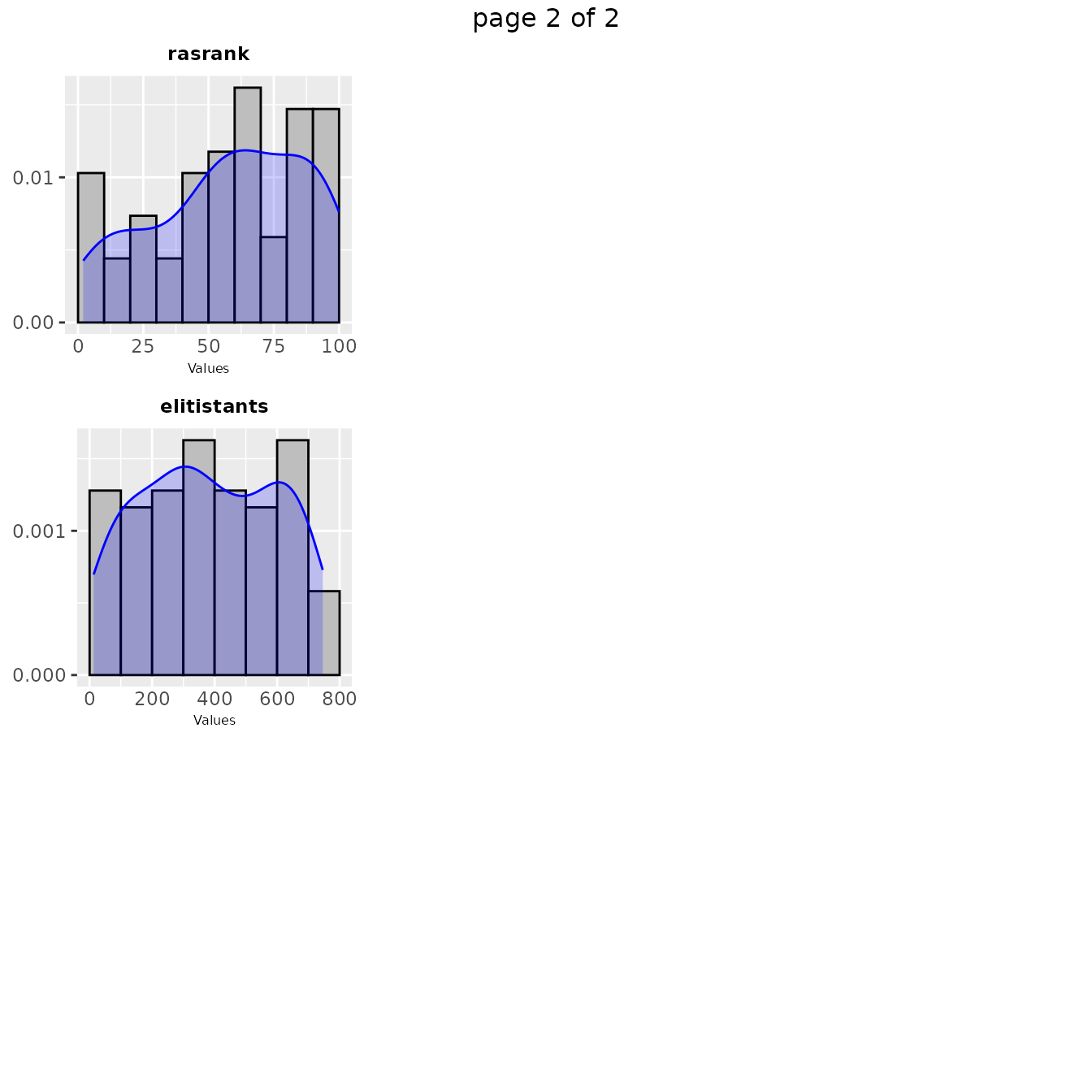If you would like to visualize the distribution of a particular set of configurations, you can pass directly a set of configurations and a parameters object in the irace format to the sampling_frequency function:  sampling_frequency(iraceResults$allConfigurations, iraceResults$parameters) The previous functions display the parameter frequency plots grouped by 9 plots, you can adjust this setting using the n argument. You can select the parameters to be displayed, with the param_names argument. You can use these plots to ge a general idea of the area of the parameter space in which the sampling performed by irace was focused. For example:  sampling_frequency(iraceResults, param_names = c("beta"))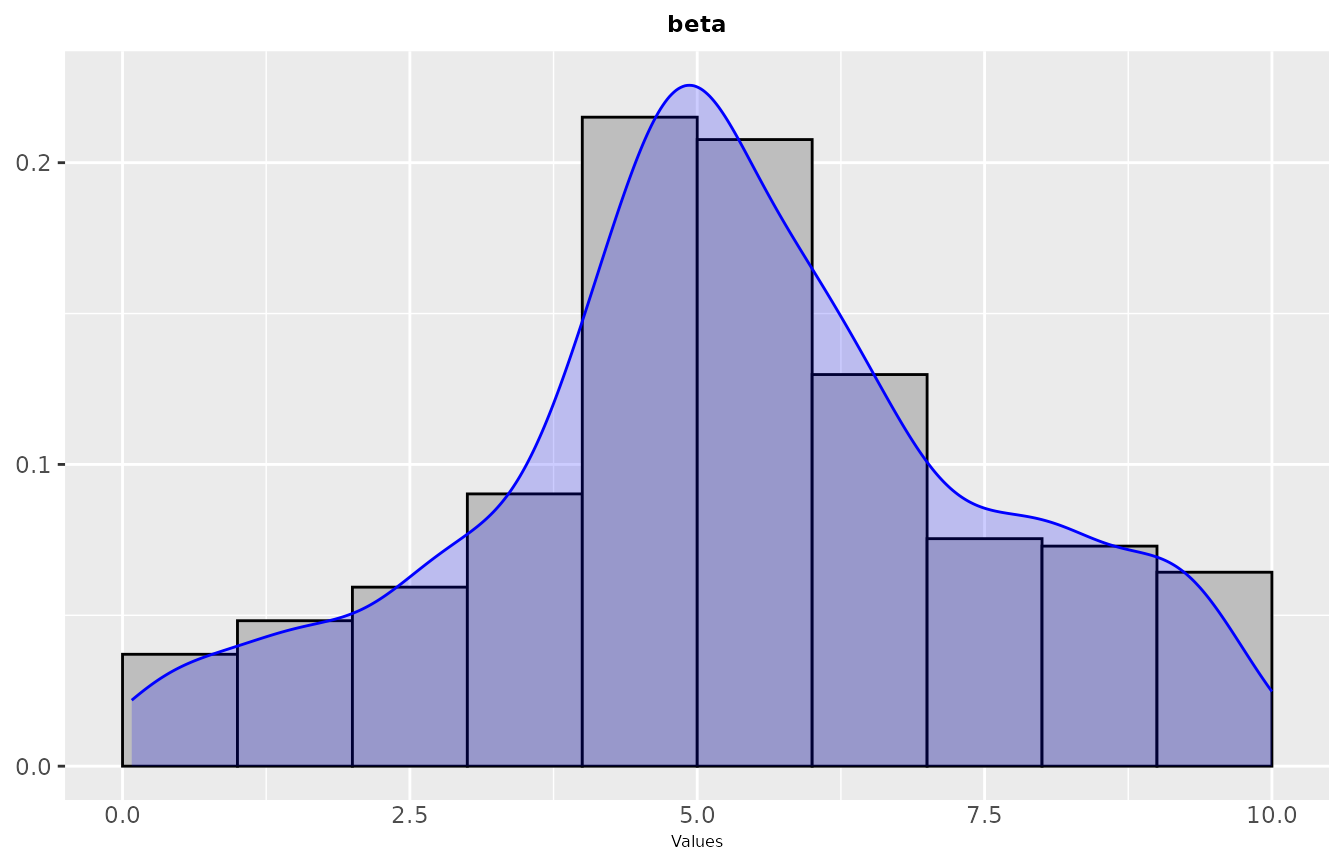If you would like to see more details, a plot showing the sampling by iteration can be obtained with the sampling_frequency_iterationfunction. This plot shows the convergence of the configuration process reflected in the parameter values sampled each iteration: sampling_frequency_iteration(iraceResults, param_name = "beta")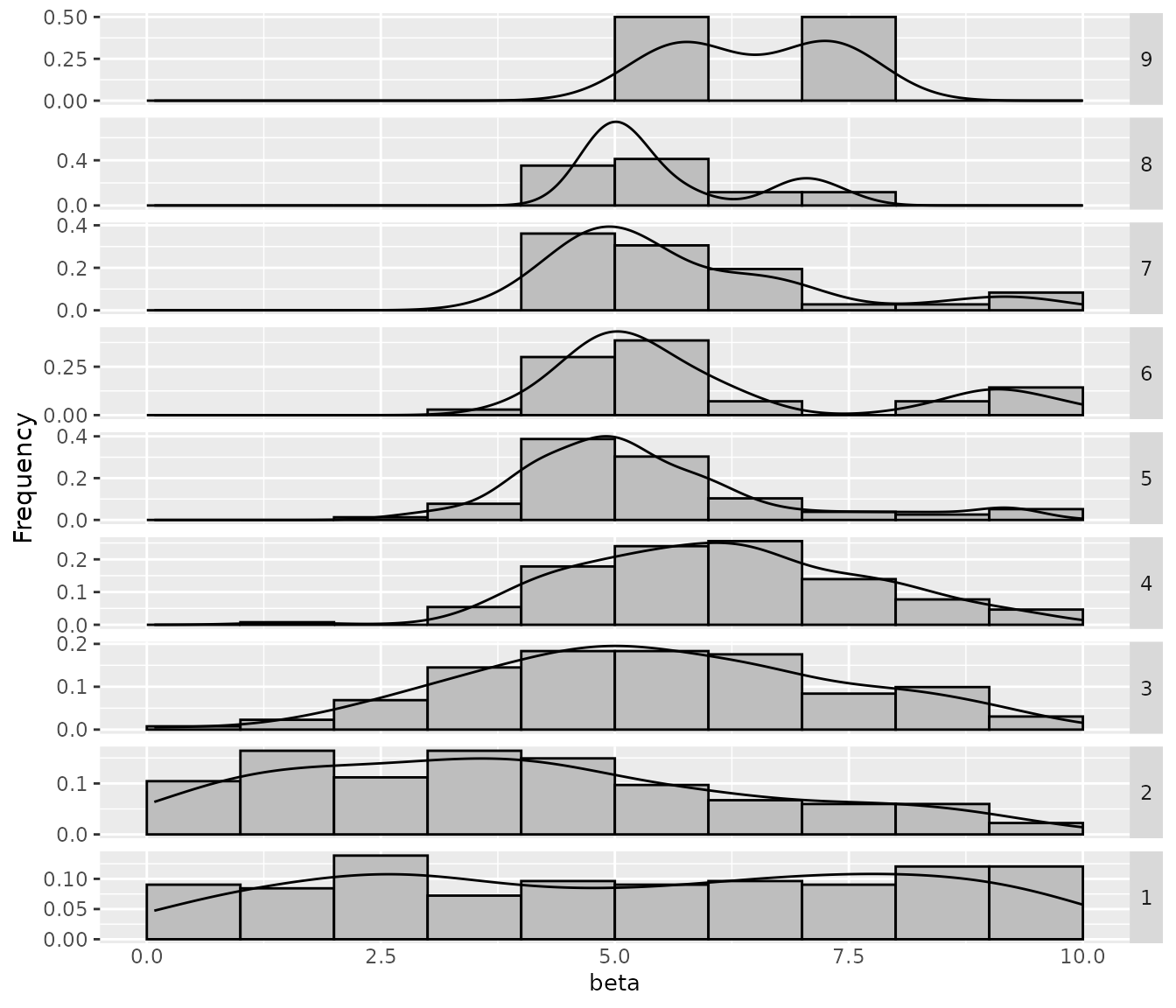In some cases, you may want to assess how the sampling frequencies of two parameters are related. You can visualize the joint sampling frequency of two parameters using the sampling_heatmap function. By default, this plot uses the elite configurations values (of all configurations), you can allow the plot to show all sampled configurations using the only_elite argument. You must select two parameters using the param_names argument, for example: sampling_heatmap(iraceResults, param_names = c("beta","alpha"))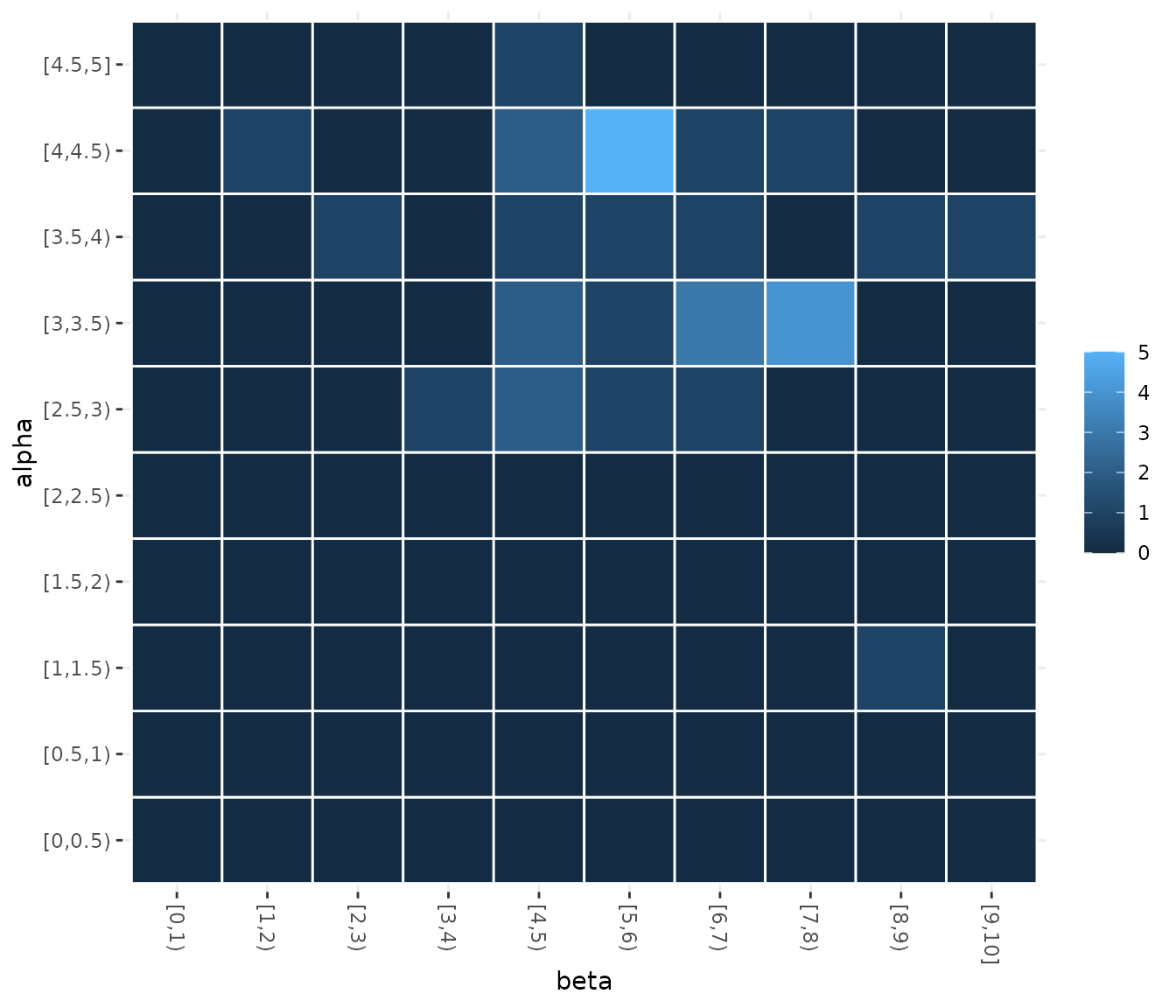You can also select the iterations from which configurations will be selected using the iterations argument. The size of the intervals considered for numerical parameters in the heatmap can be also adjusted, see the example to have more details. If you would like to display a set of configurations directly provided by you, use the sampling_heatmap2 function. In both sampling_heatmap2 and sampling_heatmap2, you can adjust the number of intervals to be displayed for numerical parameters using the sizes argument. For example, we set to 5 the number of intervals displayed for the second parameter, which is numerical, using sizes=c(0,5). The the 0 value in this argument indicates that the default interval size should be used. In this case, we set 0 for the first parameter but note that it is not possible to adjust the number of intervals for categorical or ordered parameter types. sampling_heatmap2(iraceResults$allConfigurations, iraceResults$parameters, param_names = c("localsearch","nnls"), sizes=c(0,5))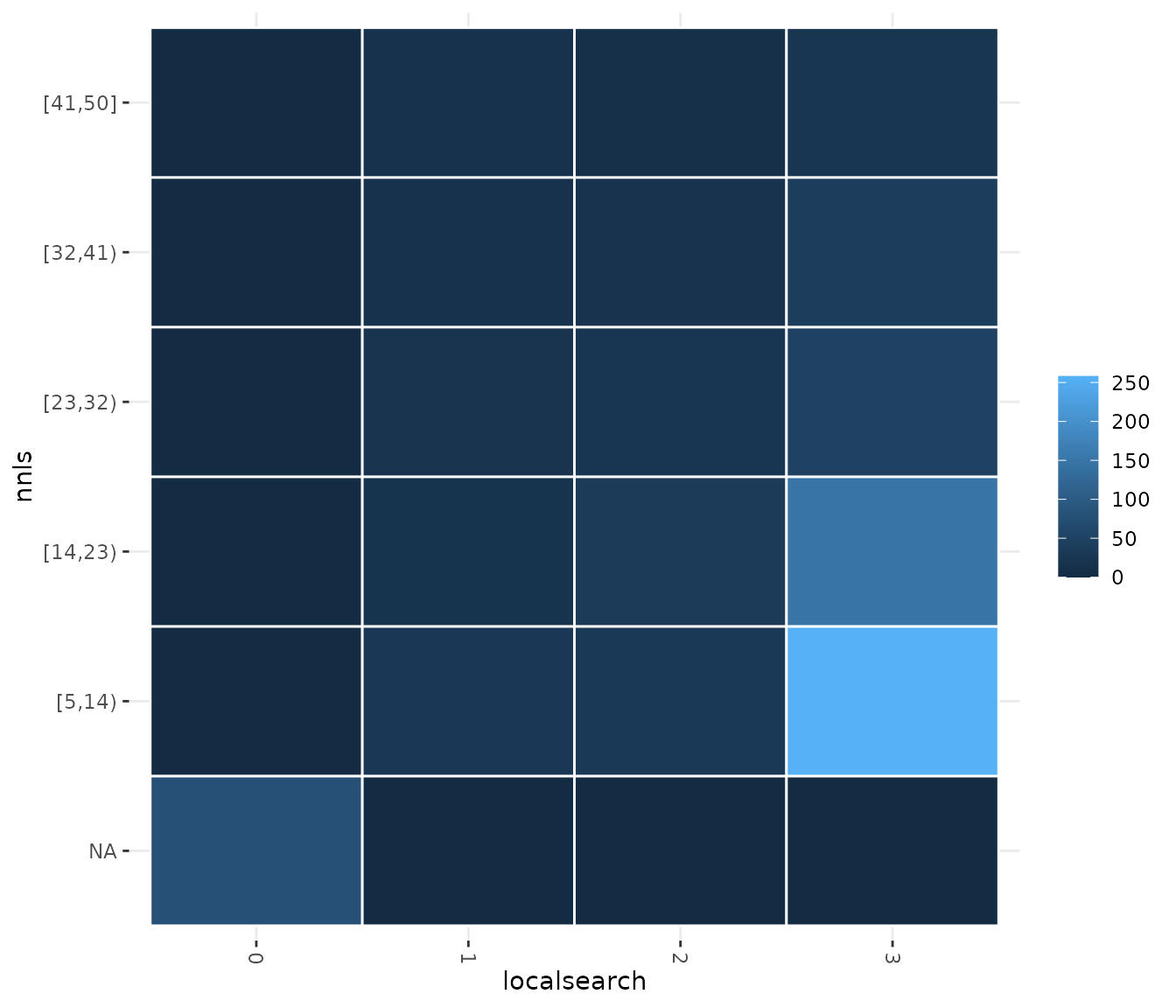### Sampling distance You may like to have a general overview of the distance of the configurations sampled across the configuration process. This can allow you to assess the convergence of the configuration process. The mean distance between the sampled configurations can be visualized using the sampling_distance function. This function compares the parameter values of all configurations and aggregates these comparisons to calculate the overall distance: sampling_distance(iraceResults, t=0.05)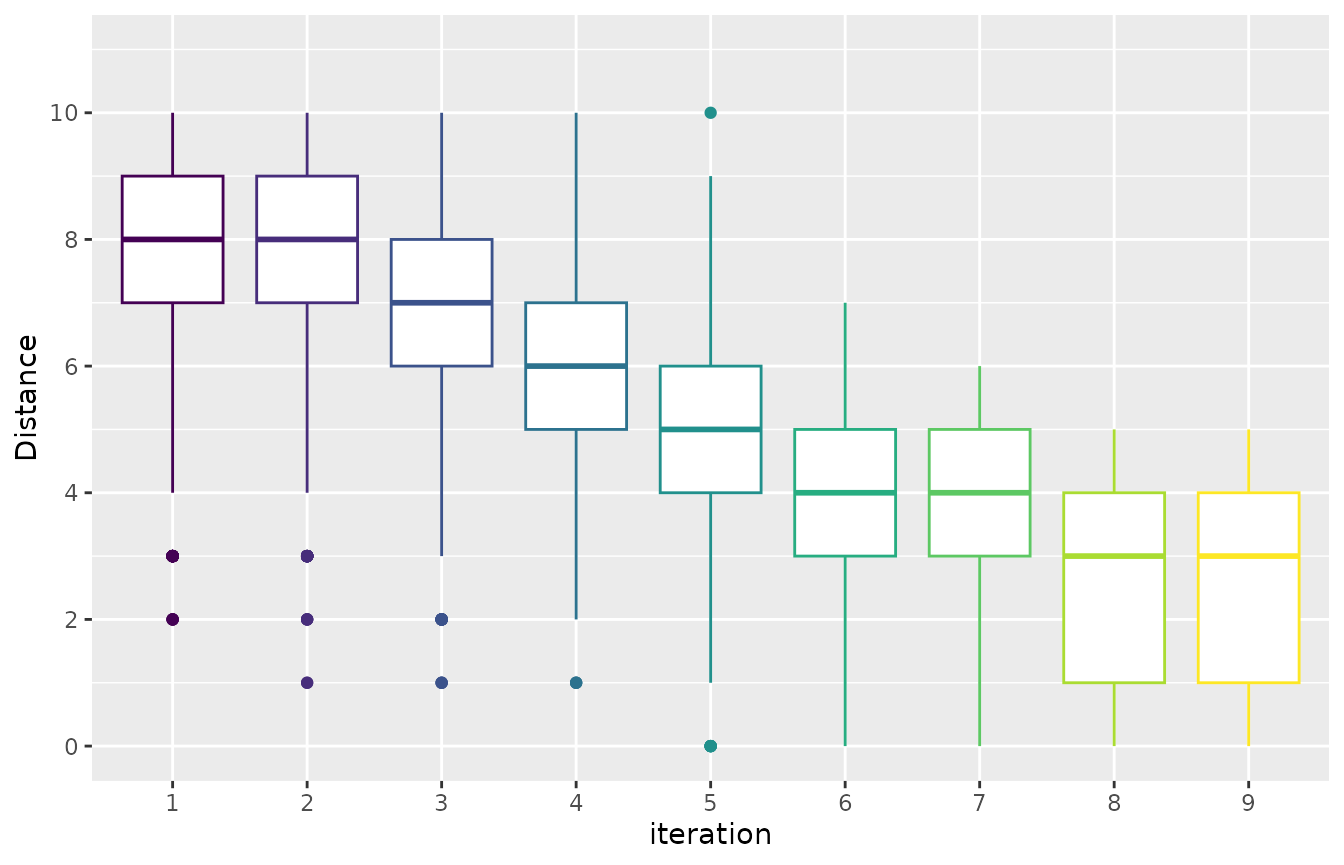Note that for categorical and ordered parameters the comparison is straightforward, but this is not the case for numerical parameters. The argument t defines a percentage used to define a domain interval to assess equality of numerical parameters. For example, if the domain of a parameter is [0,10] and t=0.1 then when comparing any value to v=2 we define an interval s=t* (upper_bound -lower_bound) = 0.1*(10-0)=1. Then all values in the interval [v-s, v+s] [1,3] will be equal to v=2. ## Visualizing Performance ### Test performance (elite configurations) When executing irace, you can enable the testing feature that will evaluate elite configurations in a set of test instances. For more details about how you can use this feature check the irace package user guide. The test performance of the evaluated configurations can be visualised using the boxplot_test function. Note that the irace execution log file includes test data (test is not enabled by default). boxplot_test(iraceResults, type="best")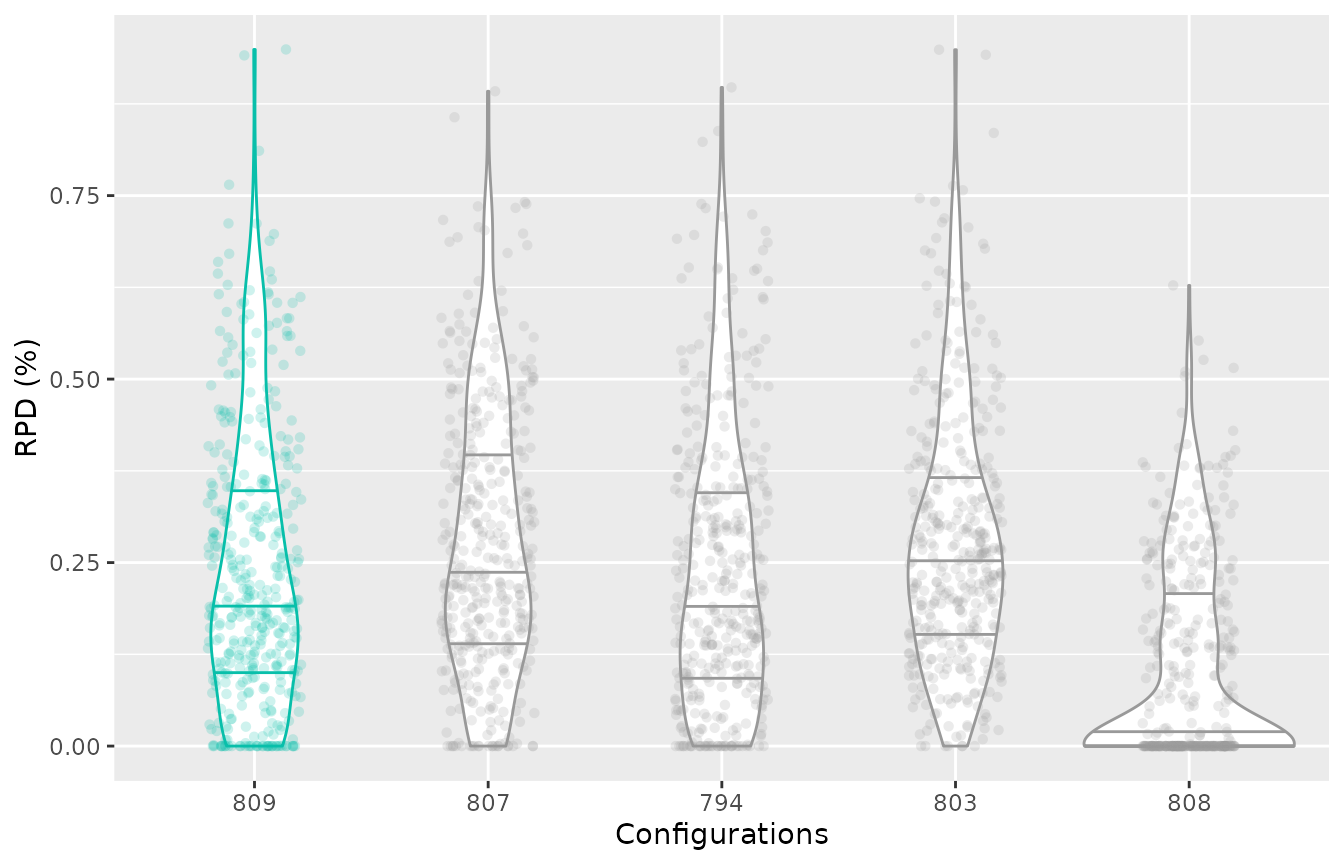This plot shows all final elite configurations evaluation on the test instance set, we can compare the performance of these configurations to select one that has the best test performance. Note that the best configuration (identified by irace) is displayed in a different color. By default, the plot displayes the relative percentage deviation of the performance of the configurations, to disable this and display the raw performance use the rpd=FALSE argument. If the irace log file includes the evaluation on the test set of the iteration elite configurations, its possible to plot the test performance of elite configurations across the iterations using the argument type="all". The best elite configuration on each iteration is displayed in different color: boxplot_test(iraceResults, type="all", show_points=FALSE)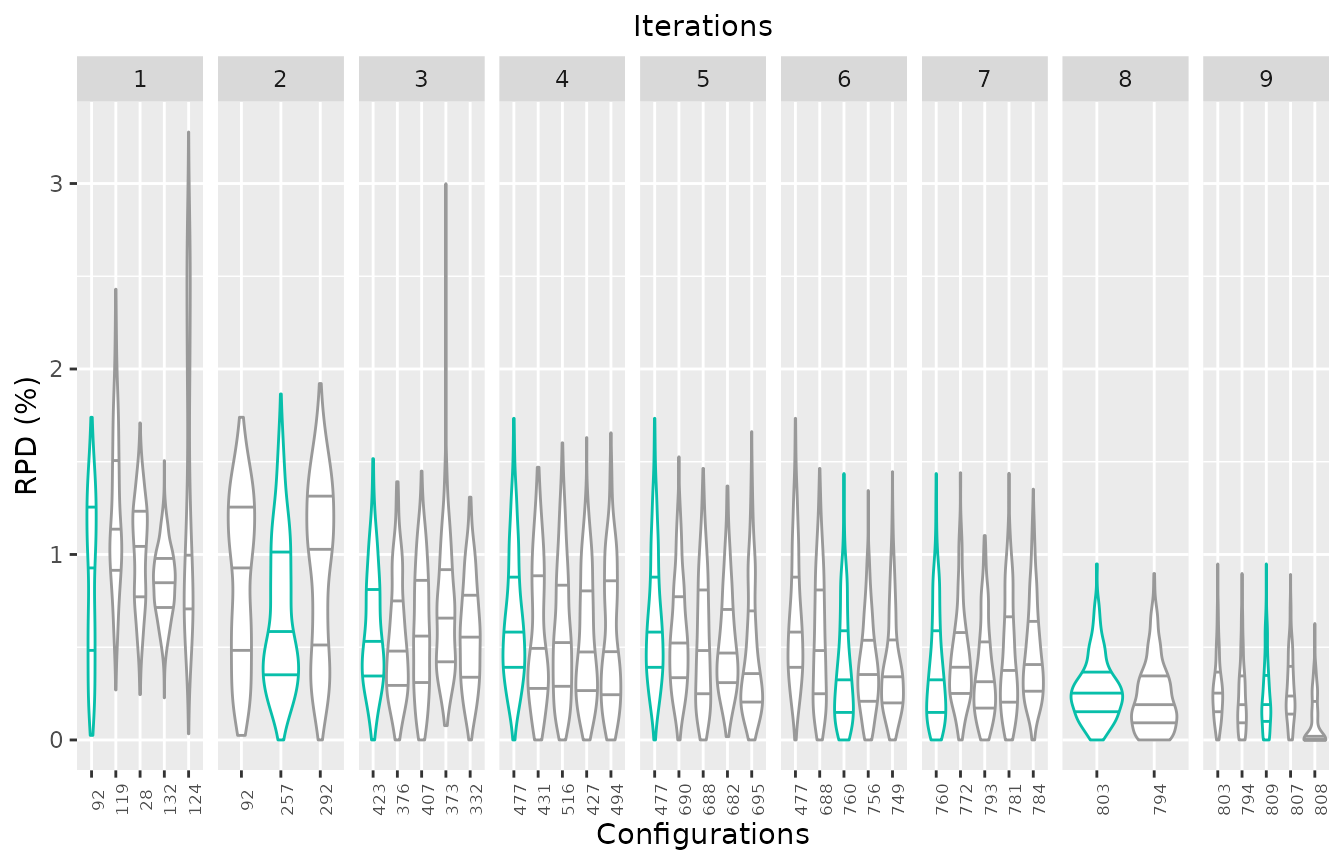This plot allows to assess the progress of the configuration process regarding the test set performance, which would be useful when dealing with heterogeneous instance sets. In these cases, good configurations across the full set can be challenging to find and it is possible that the algorithm could be mislead if instances sets are prone to introduce bias due to instance ordering. Note that in this example the elite configuration with id 808 seems to have a slightly better performance than the configuration identified as the best (id:809) by irace. It is important to note that all elite configurations are not statistically different and thus, its very possible that such situation is observed when evaluuting test performance, specially when configuring heterogeneous, difficult to balance, instance sets. If you would like further detauls about the difference in the performance of two configurations, you can use the scatter_test function. This function displays the performance of both configurations paired by instance (each point represents an instance): scatter_test(iraceResults, x_id = 808, y_id = 809, interactive=TRUE, instance_names = basename) If the plot is created using the argument interactive=TRUE you can visualize the instance name when placing the cursor over each performance point. This plot can help to identify subsets of instances in which a configuration clearly outperforms other. To further understand the difference of these two configurations the trainig data might be explored to verify if such effect holds for the training set. ### Training performance (all configurations) During the execution of irace considerable performance data is obtained in order to assess the performance of the candidate configurations. This data can be useful to understand the performance of the configured algorithm and to get insights about how to improve the configuration process. The following functions create plots of the training data in the irace log. Note that this data is obtained during the search of good configurations. Due to the racing procedure, some configurations are more evaluated than others (best configurations are more evaluated than poor performing configurations). See the irace package documentation for details. Visualizing training performance might help to obtain insights about the reasoning that followed irace when searching the parameter space, and thus it can be used to understand why irace considers certain configurations as high or low performing. To visualize the performance of the final elites as observed by irace, the boxplot_training function plots the experiments performed on these configurations. Note that this data corresponds to the performance generated during the configuration process thus, the number of instances on which the configurations were evaluated might vary between elite configurations. boxplot_training(iraceResults)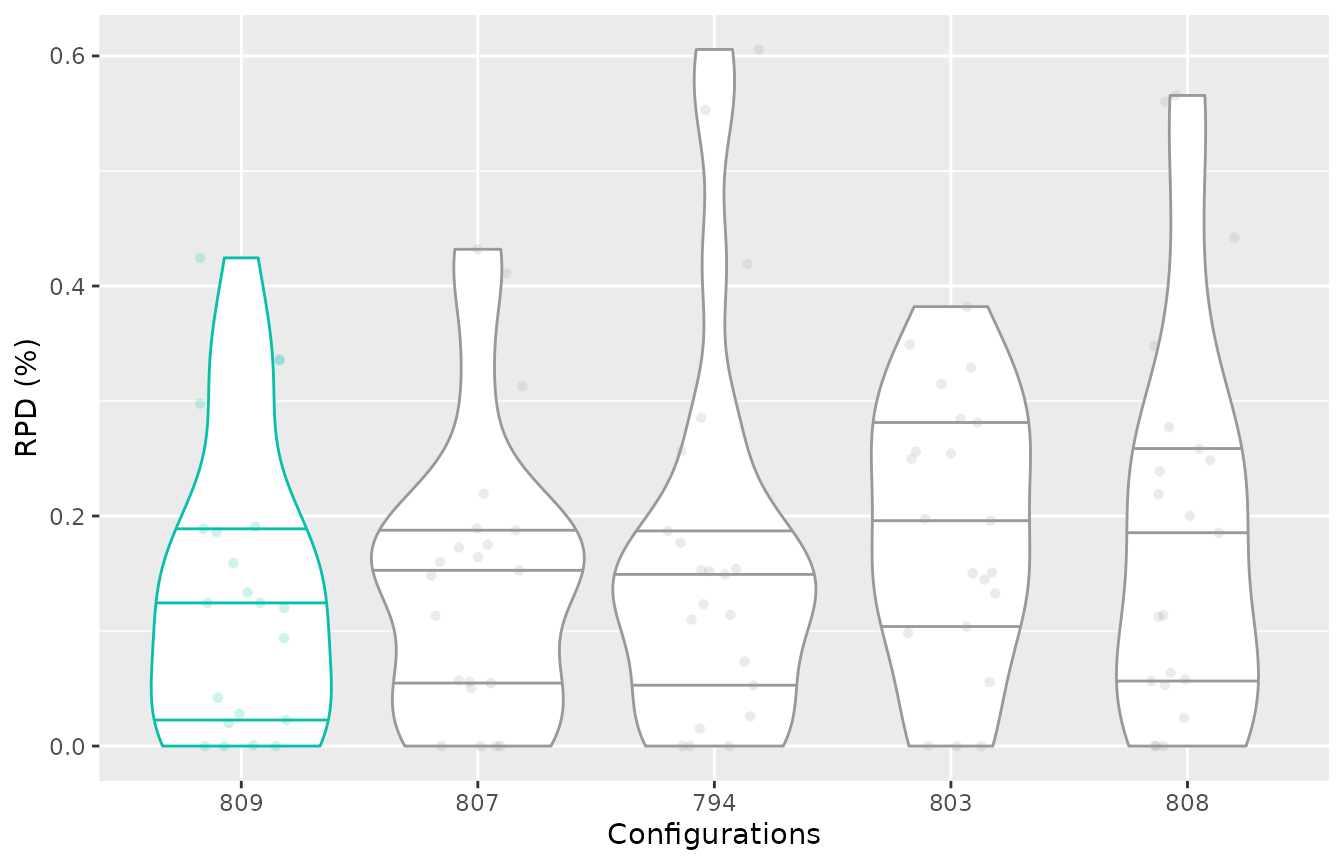You can select to display the elite configurations of a different iteration by using the iteration. In case you would like to visualize non-elite configurations you can directly provide the instance ids using the . This can be very useful to assess the performance of initial configurations provided for the configuration process for example, providing insights about why irace did not select them as final elite configurations: iteration_elites = iraceResults$iterationElites
boxplot_training(iraceResults, id_configurations=c(1, iteration_elites))To visualize the difference in the performance of two configurations you can also generate a scatter plot using the scatter_training function:

scatter_training(iraceResults, x_id = 808, y_id = 809, interactive=TRUE)

If the plot is created using the argument interactive=TRUE you can visualize the instance name when placing the cursor over each performance point.

For both functions boxplot_training and scatter_training, you can display either the relative percentage deviation (rpd=TRUE) or the raw performance (rpd=FALSE).

You can also plot the performance of configurations which was not necessarily obtained when executing irace. Check the General purpose performance section for details.

### General purpose performance

You can use the following functions to plot the performance of a selected set of configurations in an experiment matrix provided directly by you. These functions can be also useful when you would like to compare a configuration that was not generated in the configuration process. Note thar such comparison should be carefully considered as execution conditions might differ from the ones when irace was executed. Keep in mind that irace provides seeds to execute instances when configuring stochastic algorithms and also might define an execution bound when adaptive capping is active. Check the irace package user guide for details about this.

To plot the performance of a selected set of configurations in an experiment matrix, you can use the boxplot_performance function. The configurations can be selected in a vector (allElites):

boxplot_performance(iraceResults$experiments, allElites=c(800, 803,808,809))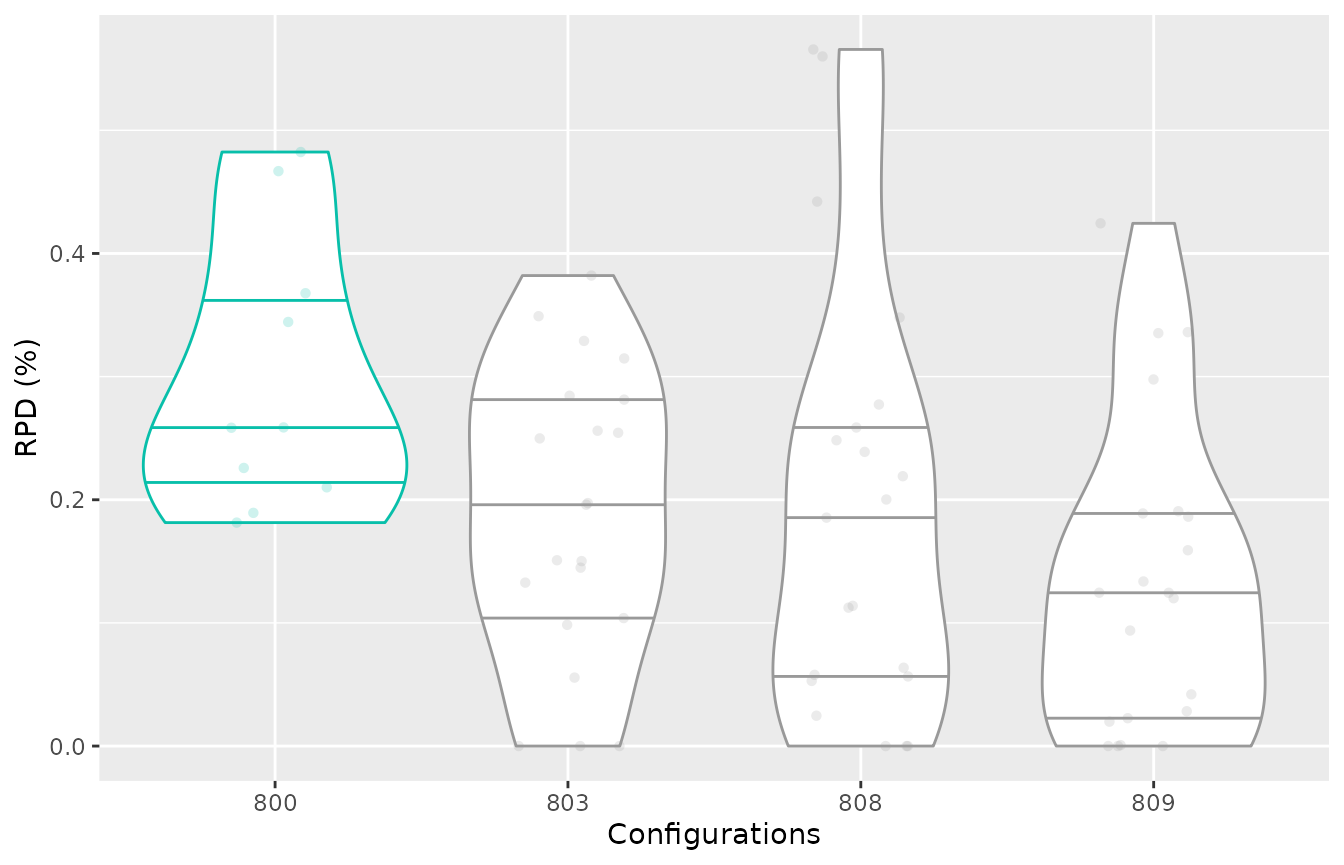The experiment matrix should be provided in the irace format, columns should have configuration ids as names (character) and rows names can be instances names (optional). If the configurations are provided in a list, then the different elements of this list will be considered as iterations. Note that this matches the iraceResults$allElites variable in the irace log. For example, to plot 3 configurations as they are assigned to 3 different iterations:

boxplot_performance(iraceResults$experiments, allElites=as.list(c(800,803,808,809)))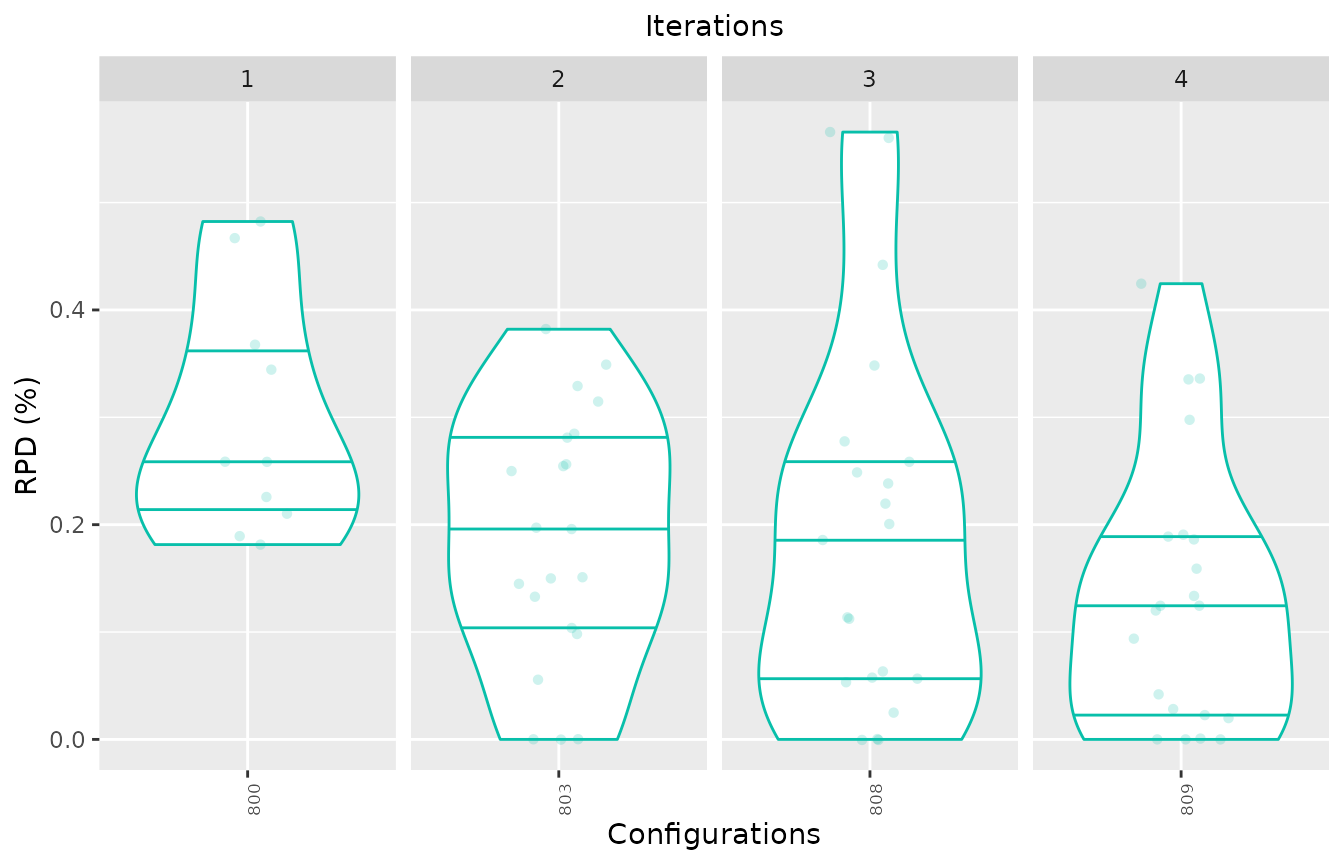Each element of a list can have more than one id (vector). You can place at the start of each vector the configuration id you want to be identified as the best one and use first_is_best = TRUE to have it displayed in the different color. Adjust the color using the best_color argument. boxplot_performance(iraceResults$experiments, allElites=list(c(803,808), c(809,800)), first_is_best = TRUE)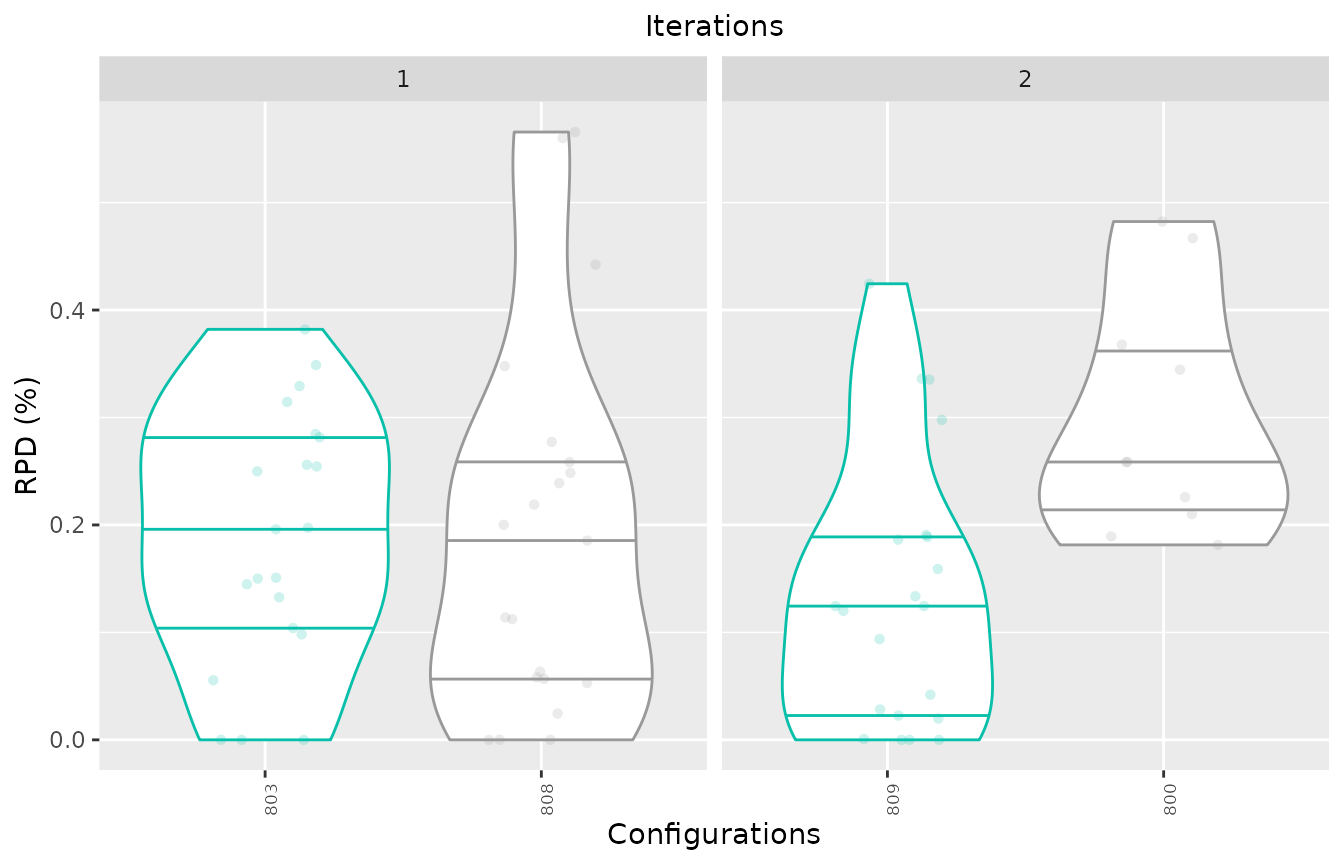If you want to further compare the performance of two configurtions, you can use the scatter_perfomance function to plot the difference between configurations:

scatter_performance(iraceResults\$experiments, x_id = 803, y_id = 809, interactive=TRUE, instance_names = basename)

If the plot is created using the argument interactive=TRUE and, the provided matrix has row names, you can visualize the instance name when placing the cursor over each performance point otherwise an instance ID is displayed. In this example, we further transform instance names using the function basename().

## Visualizing the configuration process

In some cases, it might be interesting have a general visualization for the configuration process progress. This can be generated with the plot_experiments_matrix function:

plot_experiments_matrix(iraceResults, interactive = TRUE)